# High School Physics Practicals using PSLab Device

High school physics syllabus includes rather advanced concepts in the world of Physics; namely practicals related to sound, heat, light and electric. Almost all of these practicals are done in physics labs using conventional bulky equipments. Especially the practicals related to electrical and electronics. They use bulky oscilloscopes with complex controls and multimeter with so many controls. PSLab being a sophisticated device which integrates a whole science lab into a 2” by 2” small circuit, these practicals made easy and interesting.

There are several practicals required to be completed by a student before sitting for GCE (Advanced Level) examination. This blog post points out how to perform those experiments using PSLab device and several other tools required without having to use bulky instruments.

• Calculating internal resistance and EMF of a dry cell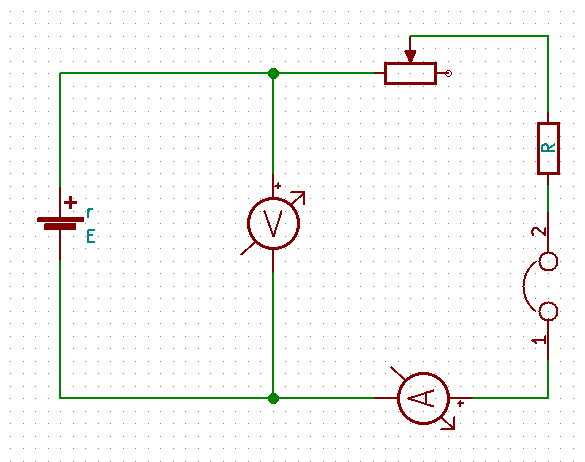This experiment includes the above mentioned circuitry. Once the basic setup is completed using;

• Dry cell
• 10K variable resistor
• 1K resistor
• Switch/Jumper

Connect the CH1 pin and GND pin of PSLab across the dry cell and CH2 pin and GND across the Ammeter instead of the Ammeter. Once the pin connection is complete, plug the PSLab device to the computer and using the desktop application voltmeter, measure the voltage from CH1 and current from voltage value read from CH2 and the known resistance value of R. Record these data against step changes in the resistance of the variable resistor starting from a high value to a low value. Once there are sufficient data as much as 10 data set, using a graph paper draw the relation between current and voltage measured according to the equation given below.

E = V + Ir

V = -rI + E

This will produce a graph with a constant negative gradient. Gradient of the graph will produce the internal resistance of the dry cell.

• I-V graphs for a semiconductor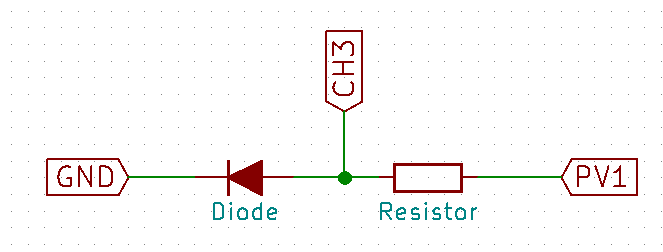This experiment will require;

• Diode
• 100 Ohms resistor

Conduct the experiment as follows once the circuit is set up. Increase the voltage provided by the PV1 pin of the PSLab by 100 mV per step and measure the voltage across diode using CH3 pin of PSLab device. Using that voltage and the supply voltage, calculate the current flowing through the resistor. Note down the readings as voltage to current pairs and plot them on a graph paper. The graph will produce the following results which is identical to common IV characteristics of a semiconductor.

Start A at zero and increase by 0.1V steps while measuring voltage and current across diode. (Image from Wikipedia)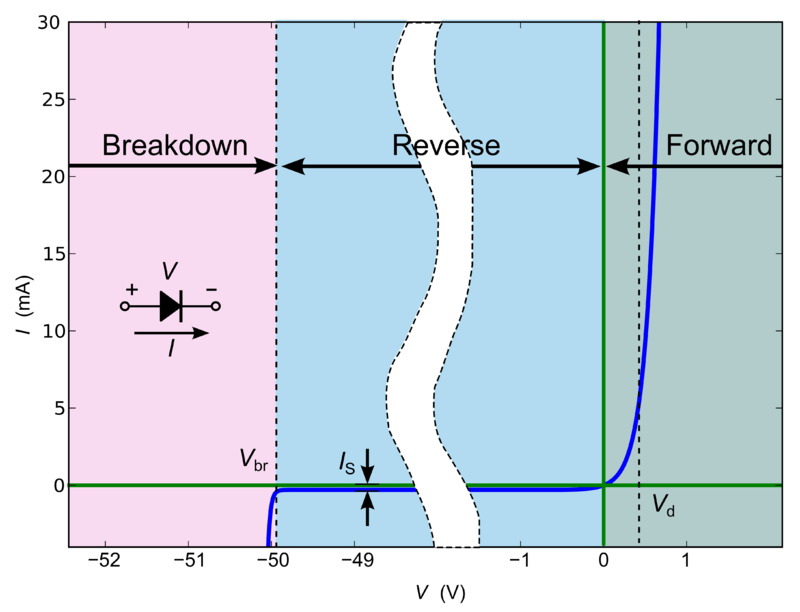Apart from this conventional experiment, PSLab provides an inbuilt experiment students and teachers can use. In the Electronics Experiments section, Diode IV characteristics experiment is configured in such a way that this experiment can be conducted very easily. The circuit configuration is the same and R value should be 1K. Once the step size is set to an appropriate resolution, START button will begin the experiment and provide with the final graph.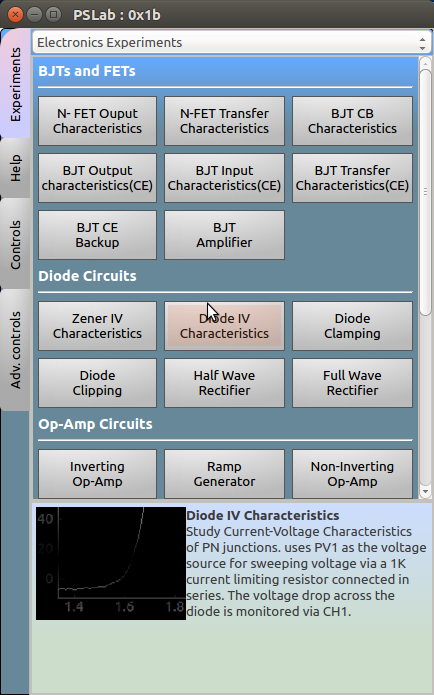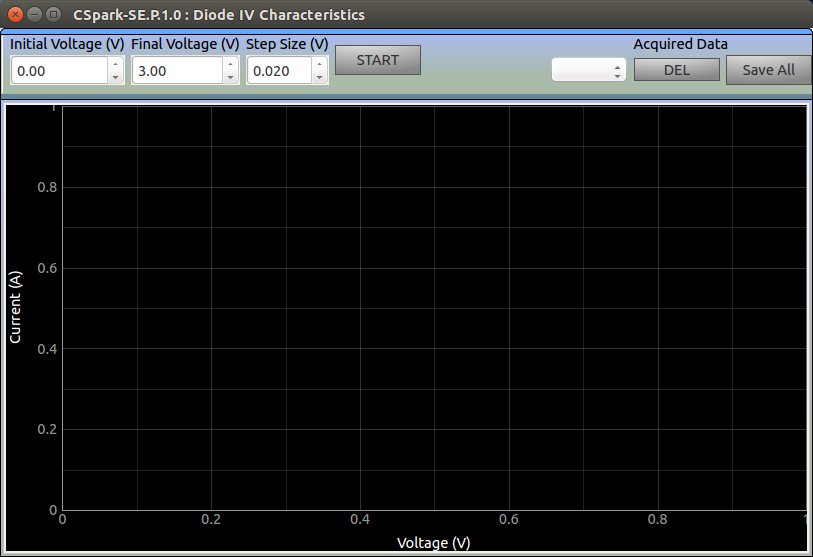• Transfer characteristics of a transistor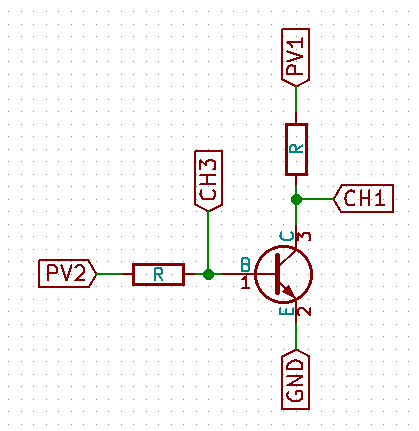Set up the above mentioned circuitry on a breadboard and connect the appropriate pins of PSLab device as mentioned. In this experiment, student need to observe the increase in current/voltage across CH1 when PV2 is varying. We need to measure CH1 parameter value against PV2 voltage value and plot these two against to obtain the transfer characteristics of a BJT.

Apart from this conventional experiment, PSLab provides an inbuilt experiment to perform this lab exercise. Using “BJT Transfer Characteristics” experiment, students and teachers can easily obtain the characteristics curves by setting step sizes and voltage values to the required pins.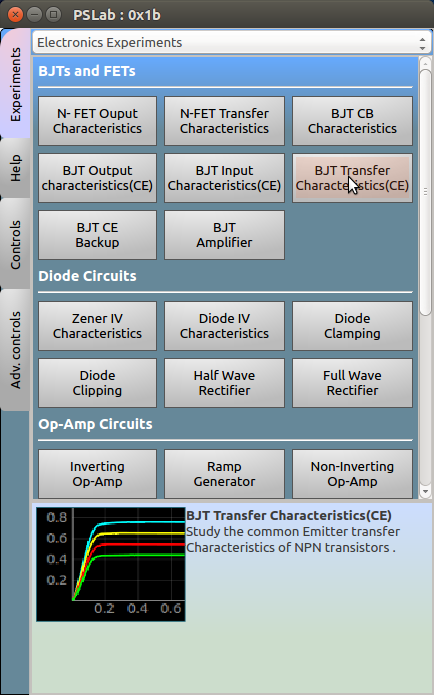• Ohm’s law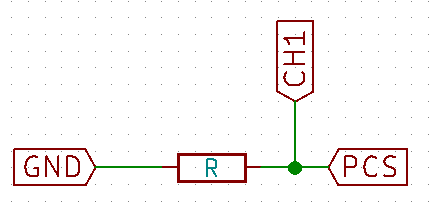Configure the following circuit and connect PCS, CH1 and GND pins of PSLab into relevant positions. This experiment is to measure voltage across the resistor against the variation in current through the circuit. Increase current provided to the circuit using PCS pin and measure voltage using CH1 pin and plot against the current. The gradient will produce the resistor value.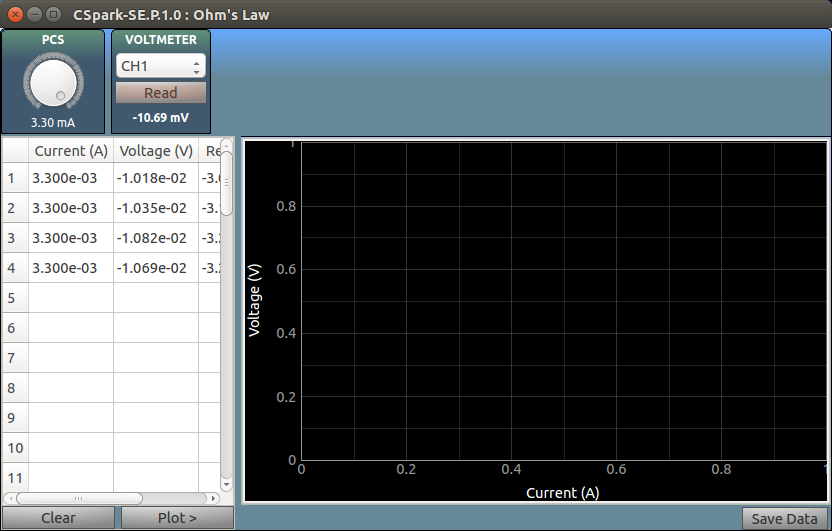In the electrical experiments section in PSLab application has a separate experiment to perform the Ohm’s Law experiment. Using this experiment, students can change the current values using the knob and record the voltage values related to that. Finally the plot can be generated which will be of a constant gradient.

• Kirchhoff’s Laws
• Voltage law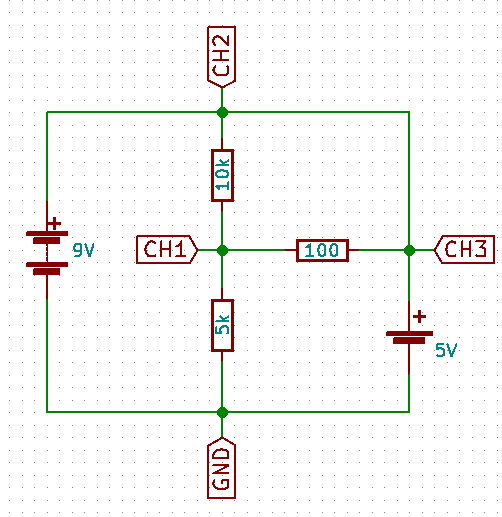Kirchhoff’s Voltage law states that the directed sum of the voltage around any closed network is zero.

This law can be proved using the following circuit with the help of PSLab device. Connect the channel pins as per the diagram and measure voltages in the three channels. They will show a relationship as the directed sum of the combination will turn into a zero.

• Current law

Kirchhoff’s current law states that at any node in a circuit, the sum of currents flowing into that node is equal to the sum of currents flowing out of that node; which is similar to the direct summation of currents in a node is zero.

Using the same circuit as above, measure the current flowing through each node using the voltage readings of the channels and known resistor values. Considering the sign of the current which is easily readable in PSLab desktop application, the summation will produce a zero.

Resources:

This site uses Akismet to reduce spam. Learn how your comment data is processed.